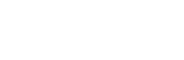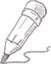#``drawROC.A <- function(T, D) {    cutpoints <- c(-Inf, sort(unique(T)), Inf)    sens <- sapply(cutpoints,                   function(c) sum(D[T>c])/sum(D))    spec <- sapply(cutpoints,                   function(c) sum((1-D)[T<=c]/sum(1-D)))    plot(1-spec, sens, type = "l")}``

drawROC.B <- function(T, D){ DD <- table(-T, D) sens <- cumsum(DD[ ,2]) / sum(DD[ ,2]) mspec <- cumsum(DD[ ,1]) / sum(DD[ ,1]) plot(mspec, sens, type="l") }

0.0000 0 3 关注作者 收藏

## 评论(0)